# Samacheer Kalvi 9th Maths Solutions Chapter 2 Real Numbers Ex 2.2

## Tamilnadu Samacheer Kalvi 9th Maths Solutions Chapter 2 Real Numbers Ex 2.2

9th Maths Exercise 2.2 Samacheer Kalvi Question 1.
Express the following rational numbers into decimal and state the kind of decimal expansion.
(i) $$\frac { 2 }{ 7 }$$
(ii) $$-5 \frac{3}{11}$$
(iii) $$\frac { 22 }{ 3 }$$
(iv) $$\frac { 327 }{ 200 }$$
Solution:
(i) $$\frac { 2 }{ 7 }$$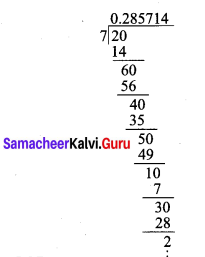$$\frac{2}{7}=0 . \overline{285714}$$
Nen-terminating and recurring

(ii) $$-5 \frac{3}{11}$$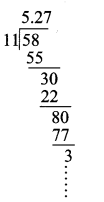$$-5 \frac{3}{11}=-5 . \overline{27}$$
Nen-terminating and recurring

(iii) $$\frac { 22 }{ 3 }$$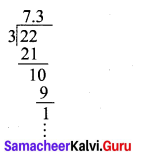$$\frac{22}{3}=7 . \overline{3}$$
Nen-terminating and recurring

(iv) $$\frac { 327 }{ 200 }$$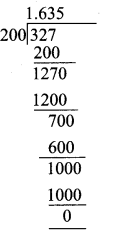$$\frac { 327 }{ 200 }$$ = 1.635, Terminating.

9th Maths Exercise 2.2 In Tamil Question 2.
Express $$\frac { 1 }{ 13 }$$ in decimal form. Find the length of the period of decimals.
Solution: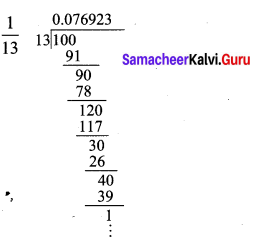$$\frac{1}{13}=0 . \overline{076923}$$ has the length of the period of decimals = 6.

9th Standard Maths Exercise 2.2 Question 3.
Express the rational number $$\frac { 1 }{ 13 }$$ in recurring decimal form by using the recurring decimal expansion of $$\frac { 1 }{ 11 }$$ . Hence write $$\frac { 71 }{ 33 }$$ in recurring decimal form.
Solution:
The recurring decimal expansion of $$\frac { 1 }{ 11 }$$ = 0.09090909…. = $$0.\overline { 09 }$$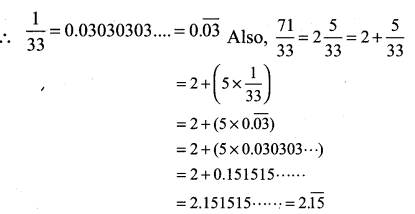Maths 9th Class Chapter 2 Real Numbers Question 4.
Express the following decimal expression into rational numbers.
(i) $$0.\overline { 24 }$$
(ii) $$2.\overline { 327 }$$
(iii) -5.132
(iv) $$3.1\overline { 7 }$$
(v) $$17.\overline { 215 }$$
(vi) $$-21.213\overline { 7 }$$
Solution:
(i) $$0.\overline { 24 }$$
Let x = $$0.\overline { 24 }$$ = 0.24242424……… ….(1)
(Here period of decimal is 2, multiply equation (1) by 100)
100x = 24.242424 ………. ….(2)
(2) – (1)
100x – x = 24.242424…. – 0.242424….
99x = 24
x = $$\frac { 24 }{ 99 }$$

(ii) $$2.\overline { 327 }$$
Let x = 2.327327327…… …………. (1)
(Here period of decimal is 3, multiply equation (1) by 1000)
1000x = 2327.327… ……………. (2)
(2) – (1)
1000x – x = 2327.327327… – 2.327327….
999x = 2325
x = $$\frac { 2325 }{ 999 }$$

(iii) -5.132
$$x=-5.132=\frac{-5132}{1000}=\frac{-1283}{250}$$

(iv) $$3.1\overline { 7 }$$
Let x = 3.1777 ……. ………… (1)
(Here the repeating decimal digit is 7, which is the second digit after the decimal point, multiply equation (1) by 10)
10x = 31.7777 …….. …………. (2)
(Now period of decimal is 1, multiply equation (2) by 10)
100x = 317.7777…….. …………….. (3)
(3) – (2)
100x – 10x = 317.777…. – 31.777….
90x = 286
$$x=\frac{286}{90}=\frac{143}{45}$$

(v) $$17.\overline { 215 }$$
Let x = 17.215215 ……. ………. (1)
1000x = 17215.215215…… …………. (2)
(2) – (1)
1000x – x = 17215.215215… – 17.215…
999x = 17198
x = $$\frac { 17198 }{ 999 }$$

(vi) $$-21.213\overline { 7 }$$
Let x = -21.2137777… ……….. (1)
10x = -212.137777…… ……….. (2)
100x = -2121.37777…… ………… (3)
1000x = -21213.77777…. ……….. (4)
10000x = 212137.77777….. ………… (5)
(Now period of decimal is 1, multiply equation (4) it by 10)
(5) – (4)
10000x – 1000x = (-212137.7777…) – (-21213.7777…)
9000x = -190924
x = –$$\frac { 190924 }{ 9000 }$$

Class 9 Maths Chapter 2 Real Numbers Question 5.
Without actual division, find which of the following rational numbers have terminating decimal expansion.
(i) $$\frac { 7 }{ 128 }$$
(ii) $$\frac { 21 }{ 15 }$$
(iii) 4$$\frac { 9 }{ 35 }$$
(iv) $$\frac { 219 }{ 2200 }$$
Solution:
(i) $$\frac { 7 }{ 128 }$$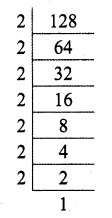So $$\frac{7}{128}=\frac{7}{2^{7} 5^{0}}$$
This of the form 4m, n ∈ W
So $$\frac { 7 }{ 128 }$$ has a terminating decimal expansion.

(ii) $$\frac { 21 }{ 15 }$$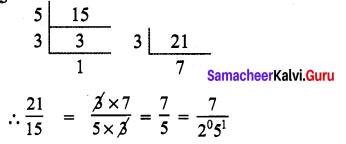So $$\frac { 21 }{ 15 }$$ has a terminating decimal expansion.

(iii) 4$$\frac { 9 }{ 35 }$$ = $$\frac { 149 }{ 35 }$$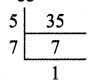$$\frac{49}{35}=\frac{149}{5^{1} 7^{1}}$$
∴ This is not of the form $$\frac{p}{5^{1} 7^{1}}$$
So 4$$\frac { 9 }{ 35 }$$ has a non-terminating recurring decimal expansion.

(iv) $$\frac { 219 }{ 2200 }$$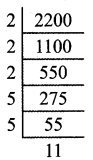$$\frac{219}{2200}=\frac{219}{2^{3} 5^{2} 11^{1}}$$
∴ This is not of the form $$\frac{p}{2^{m} 5^{n}}$$
So $$\frac { 219 }{ 2200 }$$ has a non-terminating recurring decimal expansion.